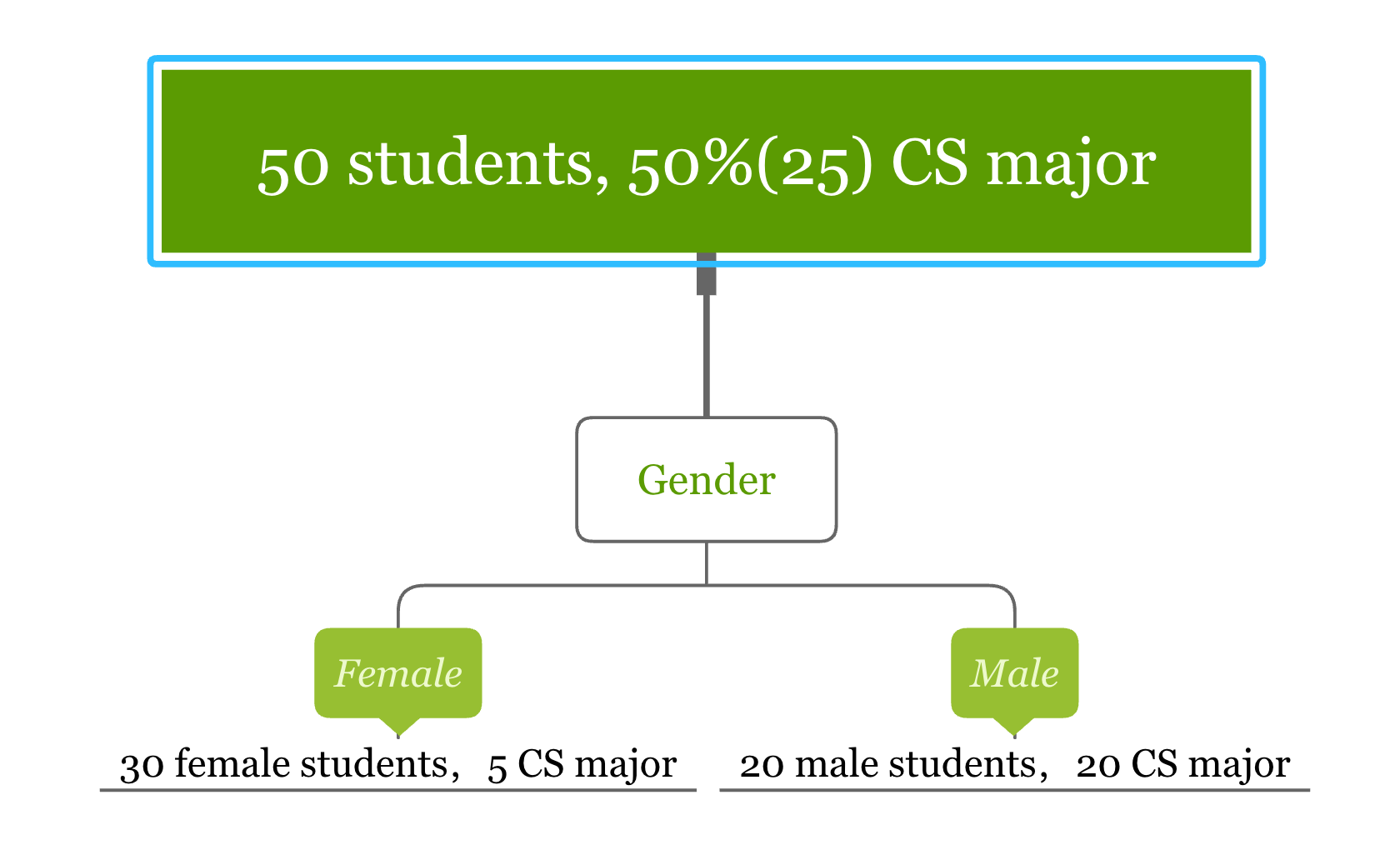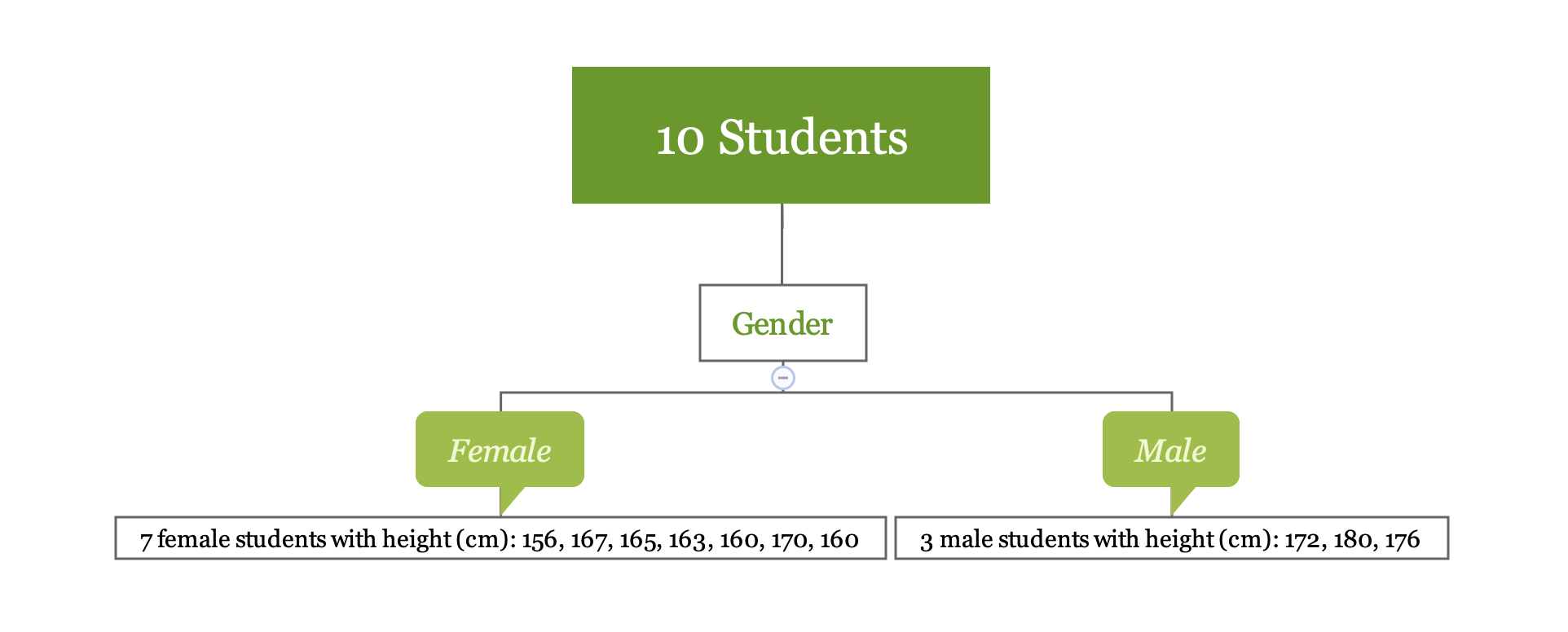# Why Tree Based Models

• Can be used for both regression and classification problems
• Do not require user to specify the form of the relationship between predictors and response
• Do not require (or if they do, very limited) data preprocessing and can handle different types of predictors (sparse, skewed, continuous, categorical, etc.)
• Robust to co-linearity
• Can handle missing data
• Many pre-built packages make implementation as easy as a button push

# A Few Definitions

• Classification tree: the outcome is discrete
• Regression tree: the outcome is continuous (e.g. the price of a house, or a patient’s length of stay in a hospital)
• Non-leaf node (or split node): the algorithm needs to decide a split at each non-leaf node (eg: $$age \leq 25$$, $$25 < age \leq 35$$, $$age > 35$$)
• Root node：the beginning node where the tree starts
• Leaf node (or Terminal node): the node stops splitting. It has the final decision of the model
• Degree of the node: the number of subtrees of a node
• Degree of the tree: the maximum degree of a node in the tree
• Pruning: remove parts of the tree that do not provide power to classify instances
• Branch (or Subtree): the whole part under a non-leaf node
• Child: the node directly after and connected to another node
• Parent: the converse notion of a child

# Splitting Criteria

The goal of splitting is to get homogenous groups.

How to define homogenous for a classification problem?

• Gini impurity (two-class problem):

$p_{1}(1-p_{1})+p_{2}(1-p_{2})$

• Entropy:

$Entropy=-plog_{2}p-(1-p)log_{2}(1-p)$

How to define homogenous for a regression problem?

• Sum of Square Error (SSE): Suppose you want to divide the data set S into two groups of S1 and S2, where the selection of S1 and S2 needs to minimize the sum of squared errors

$SSE=\Sigma_{i\in S_{1}}(y_{i}-\bar{y}_{1})^{2}+\Sigma_{i\in S_{2}}(y_{i}-\bar{y}_{2})^{2}$

# Splitting Criteria

• Gini impurity (two-class problem): $$p_{1}(1-p_{1})+p_{2}(1-p_{2})$$1. Gini impurity for “Female” = $$\frac{1}{6}\times\frac{5}{6}+\frac{5}{6}\times\frac{1}{6}=\frac{5}{18}$$
2. Gini impurity for “Male” = $$0\times1+1\times 0=0$$

The Gini impurity for the node “Gender” is the following weighted average of the above two scores:

$\frac{3}{5}\times\frac{5}{18}+\frac{2}{5}\times 0=\frac{1}{6}$

# Splitting Criteria

• Entropy: $$Entropy=-plog_{2}p-(1-p)log_{2}(1-p)$$• Root node: $$-\frac{25}{50}log_{2}\frac{25}{50}-\frac{25}{50}log_{2}\frac{25}{50}=1$$

The entropy of the split using variable “gender” can be calculated in three steps:

1. Entropy for “Female” = $$-\frac{5}{30}log_{2}\frac{5}{30}-\frac{25}{30}log_{2}\frac{25}{30}=0.65$$
2. Entropy for “Male” = $$0\times1+1\times 0=0$$
3. Entropy for the node “Gender” is the weighted average of the above two entropy numbers: $$\frac{3}{5}\times 0.65+\frac{2}{5}\times 0=0.39$$

# Splitting Criteria

• Sum of Square Error (SSE): $$SSE=\Sigma_{i\in S_{1}}(y_{i}-\bar{y}_{1})^{2}+\Sigma_{i\in S_{2}}(y_{i}-\bar{y}_{2})^{2}$$1. Root SSE: 522.9
2. SSE for “Female” is 136
3. SSE for “Male” is 32
4. SSE for splitting node “Gender” is the sum of the above two numbers which is 168

# Tree Pruning

Pruning is the process that reduces the size of decision trees. It reduces the risk of overfitting by limiting the size of the tree or removing sections of the tree that provide little power.

• Limit the size
• Minimum sample size at each node
• Maximum depth of the tree
• Maximum number of terminal nodes
• The number of variables considered for each split
• Remove branches
• cost/complexity penalty
• Error-based pruning
• Error-complexity pruning
• Minimum error pruning

Refer to this section of the book for more detail.

# Bagging Tree

A single tree is unstable, and Bootstrap aggregation (Bagged) is an ensemble method that can effectively stabilize the model.

• Steps of Bagging
1. Build a model on different bootstrap samples to form an ensemble, say $$B$$ samples
2. For a new sample, each model will give a prediction: $$\hat{f}^1(x),\hat{f}^2(x)\dots,\hat{f}^B(x)$$
3. The bagged model’s prediction is the average of all the predictions:

$\hat{f}_{avg}(x)=\frac{1}{B}\Sigma^B_{b=1}\hat{f}^b(x)$

• stabilizes the model predictions by averaging the results.
• provides more accurate predictions.
• can use out-of-bag samples to evaluate model performance.
• Computation and memory requirements
• The bagged model is difficult to explain
• Since the bagging tree uses all of the original predictors, trees are correlated, which prevents bagging from optimally reducing the variance of the predicted values.

# Random Forest

To solve one of the disadvantage of bagging tree (i.e. correlation among these trees), random forest was introduced.

• Steps of Random Forest
1. Select the number of trees, B
2. for i=1 to B do
• generate a bootstrap sample of the original data
• train a tree on this sample
• for each split do
• randomly select m（<p）predictors
• choose the best one out of the $$m$$ and partition the data
• end
• use typical tree model stopping criteria to determine when a tree is complete without pruning
3. end

1. Initialize all predictions to the sample log-odds: $$f_{i} = log \frac{\hat{p}}{1- \hat{p}}$$
• Compute predicted event probability: $$\hat{p}_i=\frac{1}{1+exp[-f_{i}(x)]}$$.
• Compute the residual (i.e. gradient): $$z_i=y_i-\hat{p}_i$$
• Compute the terminal node estimates of the Pearson residuals: $$r_i=\frac{1/n\Sigma_i^n(y_i-\hat{p}_i)}{1/n\Sigma_i^n\hat{p}_i(1-\hat{p}_i)}$$
• Update f：$$f_i=f_i+\lambda f_i^{(j)}$$